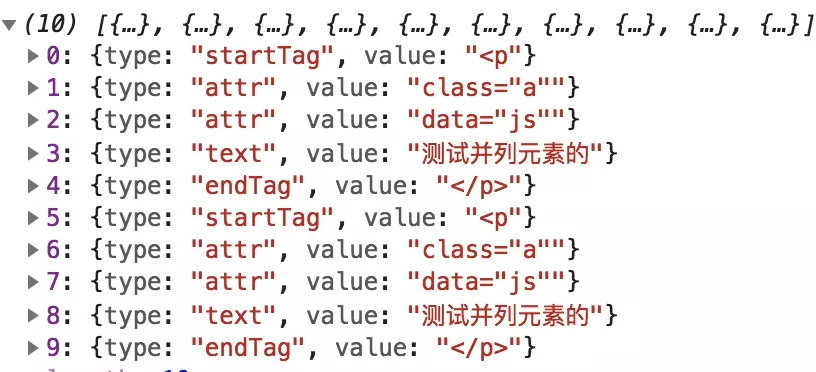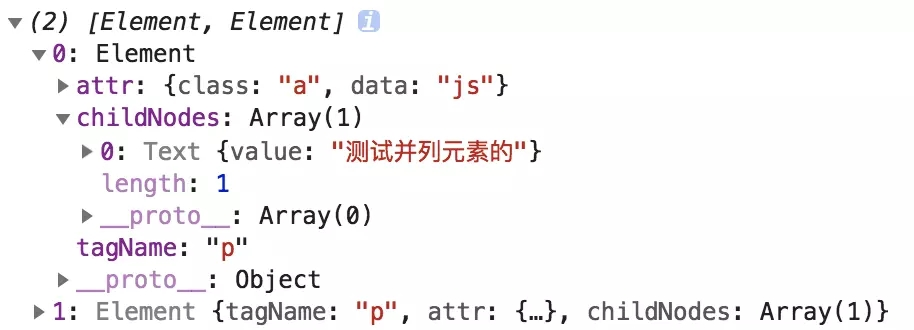# 用原生 JS 实现 innerHTML 功能实例详解

```function html(element, htmlString) {
// 1. 词法分析

// 2. 语法分析

// 3. 解释执行
}```

`测试元素`

• 开始标签：
• 属性标签：class="a"
• 文本节点：测试元素
• 结束标签：

```function HTMLLexicalParser(htmlString, tokenHandler) {
this.token = [];
this.tokens = [];
this.htmlString = htmlString
this.tokenHandler = tokenHandler
}```

• token：token 的每个字符
• tokens：存储一个个已经得到的 token
• htmlString：待处理字符串
• tokenHandler：token 处理函数，我们每得到一个 token 时，就已经可以进行流式解析

```HTMLLexicalParser.prototype.start = function(c) {
if(c === '<') {
this.token.push(c)
return this.tagState
} else {
return this.textState(c)
}
}```

start 处理的比较简单，如果是 < 字符，表示开始标签或结束标签，因此我们需要下一个字符信息才能确定到底是哪一类 token，所以返回 tagState 函数去进行再判断，否则我们就认为是文本节点，返回文本状态函数。

```HTMLLexicalParser.prototype.tagState = function(c) {
this.token.push(c)
if(c === '/') {
return this.endTagState
} else {
return this.startTagState
}
}
HTMLLexicalParser.prototype.textState = function(c) {
if(c === '<') {
this.emitToken('text', this.token.join(''))
this.token = []
return this.start(c)
} else {
this.token.push(c)
return this.textState
}
}```

emitToken 用来将产生的完整 token 存储在 tokens 中，参数是 token 类型和值。

startTagState 用来处理开始标签，这里有三种情形

• 如果接下来的字符是字母，则认定依旧处于开始标签态
• 遇到空格，则认定开始标签态结束，接下来是处理属性了
• 遇到>，同样认定为开始标签态结束，但接下来是处理新的节点信息
• endTagState用来处理结束标签，结束标签不存在属性，因此只有两种情形
• 如果接下来的字符是字母，则认定依旧处于结束标签态
• 遇到>，同样认定为结束标签态结束，但接下来是处理新的节点信息

```HTMLLexicalParser.prototype.emitToken = function(type, value) {
var res = {
type,
value
}
this.tokens.push(res)
// 流式处理
this.tokenHandler && this.tokenHandler(res)
}```
```HTMLLexicalParser.prototype.startTagState = function(c) {
if(c.match(/[a-zA-Z]/)) {
this.token.push(c.toLowerCase())
return this.startTagState
}
if(c === ' ') {
this.emitToken('startTag', this.token.join(''))
this.token = []
return this.attrState
}
if(c === '>') {
this.emitToken('startTag', this.token.join(''))
this.token = []
return this.start
}
}```
```HTMLLexicalParser.prototype.endTagState = function(c) {
if(c.match(/[a-zA-Z]/)) {
this.token.push(c.toLowerCase())
return this.endTagState
}
if(c === '>') {
this.token.push(c)
this.emitToken('endTag', this.token.join(''))
this.token = []
return this.start
}
}```

• 如果是字母、单引号、双引号、等号，则认定为依旧处于属性标签态
• 如果遇到空格，则表示属性标签态结束，接下来进入新的属性标签态
• 如果遇到>，则认定为属性标签态结束，接下来开始新的节点信息

```HTMLLexicalParser.prototype.attrState = function(c) {
if(c.match(/[a-zA-Z'"=]/)) {
this.token.push(c)
return this.attrState
}
if(c === ' ') {
this.emitToken('attr', this.token.join(''))
this.token = []
return this.attrState
}
if(c === '>') {
this.emitToken('attr', this.token.join(''))
this.token = []
return this.start
}
}```

```HTMLLexicalParser.prototype.parse = function() {
var state = this.start;
for(var c of this.htmlString.split('')) {
state = state.bind(this)(c)
}
}

HTMLLexicalParser.prototype.getOutPut = function() {
return this.tokens
}```

HTML 字符串，输出结果为```function Element(tagName) {
this.tagName = tagName
this.attr = {}
this.childNodes = []
}

function Text(value) {
this.value = value || ''
}```

• 如果是 startTag token，直接 push 一个新 element
• 如果是 endTag token，则表示当前节点处理完成，此时出栈一个节点，同时将该节点归入栈顶元素节点的 childNodes 属性，这里需要做个判断，如果出栈之后栈空了，表示整个节点处理完成，考虑到可能有平行元素，将元素 push 到 stacks。
• 如果是 attr token，直接写入栈顶元素的 attr 属性
• 如果是 text token，由于文本节点的特殊性，不存在有子节点、属性等，就认定为处理完成。这里需要做个判断，因为文本节点可能是根级别的，判断是否存在栈顶元素，如果存在直接压入栈顶元素的 childNodes 属性，不存在 push 到 stacks。

```function HTMLSyntacticalParser() {
this.stack = []
this.stacks = []
}
HTMLSyntacticalParser.prototype.getOutPut = function() {
return this.stacks
}
// 一开始搞复杂了，合理利用基本数据结构真是一件很酷炫的事
var stack = this.stack
if(token.type === 'startTag') {
stack.push(new Element(token.value.substring(1)))
} else if(token.type === 'attr') {
var t = token.value.split('='), key = t, value = t.replace(/'|"/g, '')
stack[stack.length - 1].attr[key] = value
} else if(token.type === 'text') {
if(stack.length) {
stack[stack.length - 1].childNodes.push(new Text(token.value))
} else {
this.stacks.push(new Text(token.value))
}
} else if(token.type === 'endTag') {
var parsedTag = stack.pop()
if(stack.length) {
stack[stack.length - 1].childNodes.push(parsedTag)
} else {
this.stacks.push(parsedTag)
}
}
}``````function vdomToDom(array) {
var res = []
for(let item of array) {
res.push(handleDom(item))
}
return res
}
function handleDom(item) {
if(item instanceof Element) {
var element = document.createElement(item.tagName)
for(let key in item.attr) {
element.setAttribute(key, item.attr[key])
}
if(item.childNodes.length) {
for(let i = 0; i < item.childNodes.length; i++) {
element.appendChild(handleDom(item.childNodes[i]))
}
}
return element
} else if(item instanceof Text) {
return document.createTextNode(item.value)
}
}```

```function html(element, htmlString) {
// parseHTML
var syntacticalParser = new HTMLSyntacticalParser()
var lexicalParser = new HTMLLexicalParser(htmlString, syntacticalParser.receiveInput.bind(syntacticalParser))
lexicalParser.parse()
var dom = vdomToDom(syntacticalParser.getOutPut())
var fragment = document.createDocumentFragment()
dom.forEach(item => {
fragment.appendChild(item)
})
element.appendChild(fragment)
}```

```html(document.getElementById('app'), '测试并列元素的测试并列元素的')
html(document.getElementById('app'), '测试你好呀，我测试一下没有深层元素的')
html(document.getElementById('app'), '测试一下嵌套很深的p的子元素p同级别')```

• 了解并初步实践了一下状态机
• 数据结构的魅力